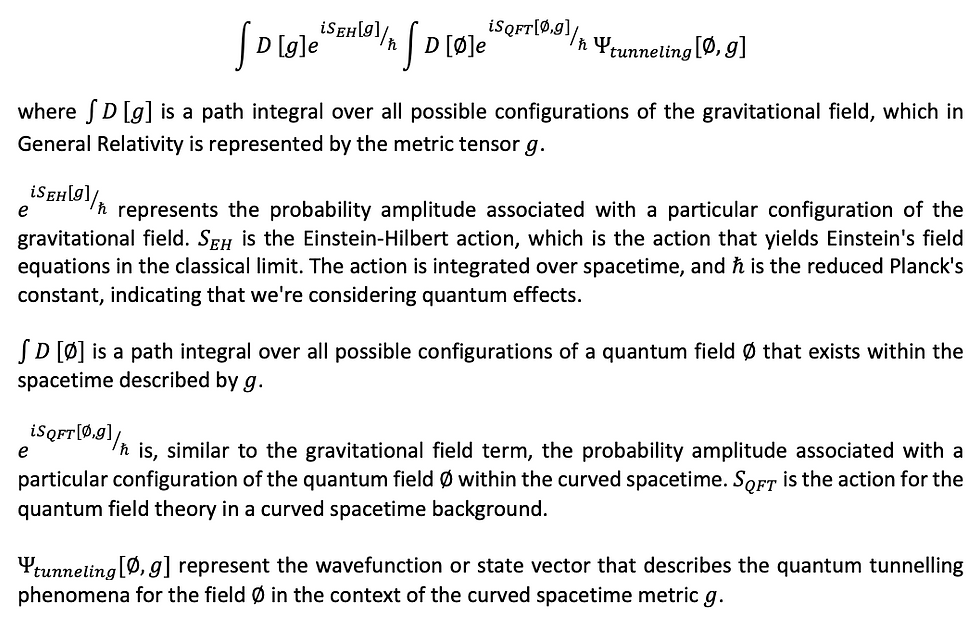top of page

# Quantum Tunnelling & Time-Space

The year 2023 seems to be one of the best years for us, as a few weeks back, we were appointed as a consultant by one of our customers for the research and development of setting up quantum computers using new technology.The Google Sycamore chip used in the creation of a time crystal. (Image credit: Google Quantum AI)

While working on the theoretical aspect of quantum computing, we have come to know that quantum tunnelling, which is also used for the operation of quantum bits (qubits) as it allows the creation of quantum gates and initialises the quantum states for quantum computations, can lead to errors in quantum computations, which necessitates the development of error correction codes and fault-tolerant quantum computing techniques.Quantum Computers. (Image credit: Pixabay/hh5800)

Quantum tunnelling is a quantum mechanical phenomenon where a particle can tunnel through a barrier that it classically could not surmount due to insufficient energy. By considering the Schrödinger equation for Quantum tunnelling, we can determine the probability amplitude of a particle's position.Schrödinger Equation for Quantum tunneling
However, during the thought process, we thought, what if we consider the effect of Time-Space on the quantification of the quantum tunnelling?Tesseract. (Image credit: Erkillers on DeviantArt)

As we all know, the theory of general relativity described by Einstein's field equations models the gravitational effects resulting from the curvature of space-time caused by mass and energy.Einstein's Field Equations

To combine these equations, we use a semi-classical approach in which quantum effects have been introduced into curved space-time by considering quantum fields in a fixed gravitational background. Such an approach resembles the Quantum Field Theory (QFT) in curved space-time.Quantum Tunnelling & Space-Time

Further, the path integral over the space-time metrics g represents a sum over the classical approach to quantum gravity, where we consider all possible space-time geometries. However, the path integral over the quantum field ϕ represents a quantum field theory in curved space-time. Furthermore, the tunnelling wave function Ψtunneling​[ϕ,g] shall be an innovative addition representing quantum tunnelling phenomena in the curved space-time.

Please note that the above mentioned equations are derived under certain assumptions and simplifications and for specific applications of quantum tunnelling. An exact derivation would likely require complex mathematical techniques from quantum field theory, general relativity, and potentially string theory or loop quantum gravity, depending on our approach to quantum gravity. Further, the path integrals for the calculation of tunnelling wave function in curved space-time require a high number of approximations.